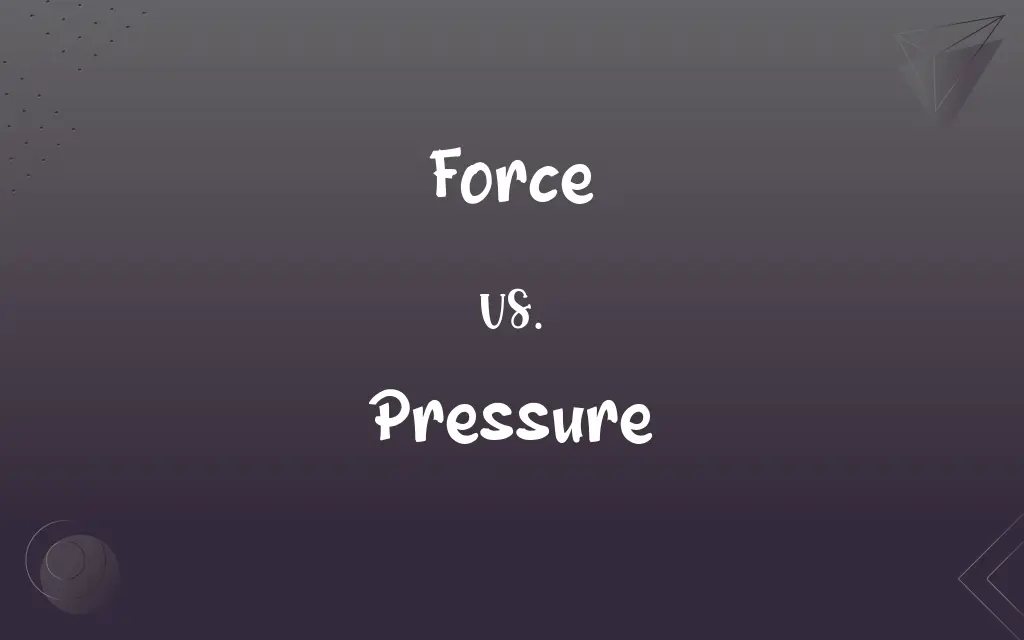# Force vs. Pressure: What's the Difference?Edited by Huma Saeed || By Sumera Saeed || Updated on October 25, 2023
Force is a push or pull acting on an object, while pressure is the force applied per unit area.## Key Differences

Force and pressure are fundamental concepts in physics, often misunderstood as interchangeable. Force, by its definition, refers to any interaction that changes the motion of an object. It can either push it, pull it, or cause some form of movement or deformation.
On the other hand, pressure describes how force is distributed over a specific area. While force can be direct and tangible, pressure gives a more concentrated picture, illustrating how much force is acting on each unit area of a surface.
It’s also important to recognize the units in which both are measured. Force is typically measured in Newtons (N) in the International System of Units. Pressure, because it’s force per unit area, is usually measured in Pascals (Pa), which translates to one Newton per square meter (N/m²).
Another distinguishing factor between force and pressure is their application in real-life scenarios. For instance, when you push a wall, you exert force. However, when you press the sharp end of a nail against a surface, the area over which the force is applied is small, leading to high pressure.

## Comparison Chart

### Definition

A push or pull on an object
Force applied per unit area

### Units

Newtons (N)
Pascals (Pa) or N/m²

### Physical Representation

Direct interaction causing movement
Distribution of force over a surface

### Nature

Can be decomposed into components
Scalar quantity

### Dependence

Independent of area
Directly depends on area and force

## Force and Pressure Definitions

#### Force

Interaction causing a change in motion.
The force of the wind pushed the tree over.

#### Pressure

Force acting on a specific area.
The pressure at the bottom of the ocean is much greater than at the surface.

#### Force

Influence causing an object to accelerate.
The force exerted by the engine moves the car.

#### Pressure

Intensity of force distributed over a surface.
The pressure of the gas inside the balloon causes it to expand.

#### Force

A push or pull on an object.
He applied force to open the jammed door.

#### Pressure

Scalar measure of force's concentration.
The tight shoes exerted too much pressure on her feet.

#### Force

Vector quantity with both magnitude and direction.
Gravity is a force pulling objects toward the Earth's center.

#### Pressure

Resultant of perpendicular force on a surface.
Atmospheric pressure decreases with altitude.

#### Force

Mechanical energy causing deformation or movement.
The force of the hammer drove the nail into the wood.

#### Pressure

Influence or stress applied to a system.
There's a lot of pressure in the boiler; it might burst.

#### Force

The capacity to do work or cause physical change; energy, strength, or active power
The force of an explosion.

#### Pressure

The act of pressing.

## FAQs

#### What's the relationship between force and acceleration?

According to Newton's second law, force equals mass times acceleration.

#### Why do pointed objects like needles exert more pressure?

Because the force is concentrated over a smaller area, resulting in higher pressure.

#### Is force always visible?

No, some forces like gravity or magnetic forces are invisible.

#### Can pressure exist without force?

No, pressure is a result of force applied over an area.

#### Can force exist without contact?

Yes, forces like gravity or magnetism can act at a distance.

#### What instrument measures atmospheric pressure?

A barometer measures atmospheric pressure.

#### Can multiple forces act on a single object?

Yes, and the net effect is determined by their vector sum.

#### Is pressure always compressive?

Typically yes, but in certain contexts, like tension in a membrane, it can be considered tensile.

#### What happens to pressure when force is distributed over a larger area?

Pressure decreases when force is spread over a larger area.

#### How do pressure cookers work?

They increase the internal pressure, which raises the boiling point, cooking food faster.

#### How is force related to momentum?

Force is the rate of change of momentum.

#### Is pressure a force?

No, pressure is the force per unit area.

#### How is pressure related to depth in a fluid?

Pressure increases with depth in a fluid due to the weight of the fluid above.

#### What is the fundamental force behind friction?

Friction arises from electromagnetic forces between atoms and molecules.

#### How is weight related to force?

Weight is the gravitational force acting on an object.

#### Is force a scalar or vector?

Force is a vector, having both magnitude and direction.

#### Can forces be added together?

Yes, forces can be combined using vector addition.

#### Does temperature affect pressure in a gas?

Yes, increasing temperature generally increases pressure if the volume is constant.

#### Are there fundamental forces in nature?

Yes, there are four fundamental forces: gravitational, electromagnetic, strong nuclear, and weak nuclear.

#### How does altitude affect atmospheric pressure?

Atmospheric pressure decreases with increasing altitude.